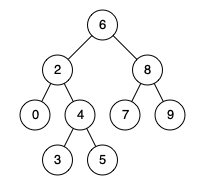# 二叉搜索树的最近公共祖先 Lowest Common Ancestor of a Binary Search Tree

lowest-common-ancestor-of-a-binary-search-tree

## 题目

Given a binary search tree (BST), find the lowest common ancestor (LCA) of two given nodes in the BST.

According to the definition of LCA on Wikipedia: “The lowest common ancestor is defined between two nodes p and q as the lowest node in T that has both p and q as descendants (where we allow a node to be a descendant of itself).”

Given binary search tree: root = [6,2,8,0,4,7,9,null,null,3,5]Example 1:

``````Input: root = [6,2,8,0,4,7,9,null,null,3,5], p = 2, q = 8
Output: 6
Explanation: The LCA of nodes 2 and 8 is 6.
``````

Example 2:

``````Input: root = [6,2,8,0,4,7,9,null,null,3,5], p = 2, q = 4
Output: 2
Explanation: The LCA of nodes 2 and 4 is 2, since a node can be a descendant of itself according to the LCA definition.
``````

Note:

``````All of the nodes' values will be unique.
p and q are different and both values will exist in the BST.
``````

https://leetcode-cn.com/problems/lowest-common-ancestor-of-a-binary-search-tree/

## 思路&题解

``````/**
* Definition for a binary tree node.
* public class TreeNode {
*     int val;
*     TreeNode left;
*     TreeNode right;
*     TreeNode(int x) { val = x; }
* }
*/
public TreeNode lowestCommonAncestor(TreeNode root, TreeNode p, TreeNode q) {
TreeNode tmp = root;
while(tmp!=null){
if(tmp.val > p.val && tmp.val > q.val){
tmp = tmp.left;
} else if(tmp.val < p.val && tmp.val < q.val) {
tmp = tmp.right;
} else if(tmp.val == p.val || tmp.val == q.val) {
return tmp;
} else {
return tmp;
}
}
return tmp;
}
``````